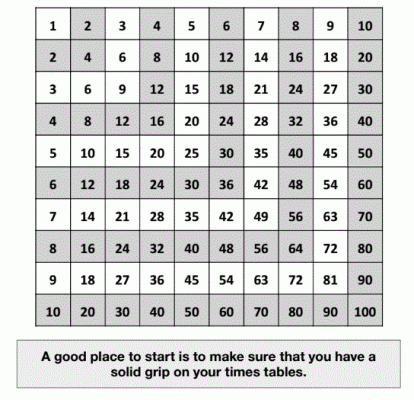# Learn How to Multiply – Methods and Examples

Do you need help with learning how to multiply? Multiplication is something that every person needs to learn. It is a vital skill to have – not just for school, but for everyday life.

Many people find it easy when multiplying smaller numbers. But what happens when you have two large numbers to multiply? Would you be able to work out this more complex calculation?
This blog will outline three different multiplication methods to master how to multiply ANY number:

• Short multiplication
• Long multiplication
• The grid method

Generally, most people find one of the three easier than the rest. I find the grid method the easiest, which can be used for any numbers – large or small!

Within this blog, and a FREE video resource, you will learn:

• How to multiply big numbers;
• How to work out long multiplication;
• How to multiply whole numbers.## LEARN HOW TO MULTIPLY VIDEO

Watch our video on how to multiply. Our Careervidz channel on Youtube is a highly popular educational channel, which has lots of videos ranging from Maths and English, and ventures out into Science and History!

Subscribe to our channel to be updated with regular uploads of our educational videos!

## MULTIPLICATION WORKSHEETS

We have created three multiplication worksheets to help you learn how to multiply. Use these, alongside our multiplication video, to become an expert in multiplying numbers!

Short Multiplication

Calculate 4258 x 5
STEP 1 = Begin by writing the smaller number underneath the larger number. Remember, you need to pay careful attention to your columns! Both last numbers need to end in the units column.STEP 2 = Multiply the ‘5’ by the number in the units column.• 8 x 5 = 40

Place the ‘0’ in the units column, and the ‘4’ in the tens column. You will need this number later!

STEP 3 = Multiply the ‘5’ by the number in the tens column.• 5 x 5 = 25
• 25 + 4 = 29

Place the ‘9’ in the tens column, and the ‘2’ in the hundreds column. You will need this number later!

STEP 4 = Multiply the ‘5’ by the number in the hundreds column.• 5 x 2 = 10
• 10 + 2 = 12

Place the ‘2’ in the hundreds column, and the ‘1’ in the thousands column. You will need this number later!

STEP 5 = Multiply the ‘5’ by the number in the thousands column.• 5 x 4 = 20
• 20 + 1 = 21

We have now used all of the numbers of the large number, so the number ‘21’ would just be placed in the answer as shown.

So, 4258 x 5 = 21,290

Long Multiplication

Calculate 159 x 35

STEP 1 = Begin by writing the smaller number underneath the larger number. Remember, you need to pay careful attention to your columns! Both last numbers need to end in the units column.STEP 2 = Multiply the ‘5’ by the number in the units column.• 5 x 9 = 45

Place the ‘5’ in the units column, and the ‘4’ in the tens column. You will need this number later!

STEP 3 = Multiply the ‘5’ by the number in the tens column.• 5 x 5 = 25
• 25 + 4 = 29

Place the ‘9’ in the tens column, and the ‘2’ in the hundreds column. You will need this number later!

STEP 4 = Multiply the ‘5’ by the number in the hundreds column.• 5 x 1 = 5
• 5 + 2 = 7

STEP 5 = Next, you need to multiply the 3 by the numbers in the top number. However, the ‘3’ is represent ‘tens’, so you need to multiply each number by ‘30’.

Multiply the ‘30’ by the number in the units column.• 30 x 9 = 270

Place the 270 directly under the sum we have just worked out (795).

STEP 6 = Multiply the ‘30’ by the number in the tens column.• 30 x 50 = 1,500

Place the 1,500 directly under the sum we have just worked out (270).

STEP 7 = Multiply the ‘30’ by the number in the hundreds column.• 30 x 100 = 3,000

Place the 3,000 directly under the sum we have just worked out (1,500).

STEP 8 = Add up all of the sums.So, 159 x 35 = 5,565

The Grid Method

Calculate 689 x 36

Step 1 = First of all, you want to break the numbers down into what they’re representing:Step 2 = Next, place these numbers into a grid, like so:Step 3 = Now, multiply the columns with the rows.Step 4 = Finally, add up all of numbers you have just filled in, in your table.• 18,000 + 2,400 + 270 = 20,670
• 3,600 + 480 + 54 = 4,134
• 20,670 + 4,134 = 24,804

So, 689 x 36 = 20,804

## MULTIPLICATION AND OTHER USEFUL RESOURCES

Click on the link for more of my other useful maths resources, guaranteed to improve your maths skills and knowledge.

This resource is packed full of maths videos, and each video focuses on a certain aspect of maths, including:

• How to multiply
• Long division
• Mean, mode, median and range
• BIDMAS
• Area, perimeter and volume
• Fractions, decimals and percentages and many more!